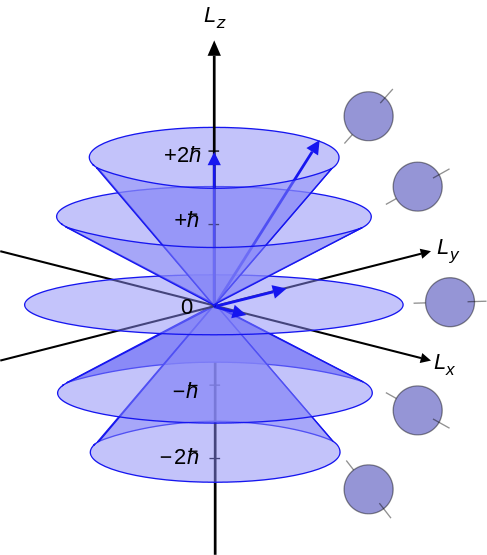10.3: Coupling of Angular Momenta

•• Contributed by Jack Simons
• Professor Emeritus and Henry Eyring Scientist (Chemistry) at University of Utah

Vector Coupling

Given two angular momenta (of any kind) $$\textbf{L}_1 \text{ and } \textbf{L}_2,$$ when one generates states that are eigenstates of their vector sum $$L= L_1+L_2$$, one can obtain $$L$$ values of

$L_1+L_2, L_1+L_2-1, ...|L_1-L_2|.$

This can apply to two electrons for which the total spin S can be 1 or 0 as illustrated in detail above, or to a p and a d orbital for which the total orbital angular momentum L can be 3, 2, or 1. Thus for a $$p^1d^1$$ electronic configuration, $$^3F, ^1F, ^3D, ^1D, ^3P, \text{and} ^1P$$ energy levels (and corresponding wavefunctions) arise. Here the term symbols are specified as the spin degeneracy (2S+1) and the letter that is associated with the L-value. If spin-orbit coupling is present, the $$^3F$$ level further splits into J= 4, 3, and 2 levels which are denoted $$^3F_4, ^3F_3, \text{ and } ^3F_2.$$

This simple "vector coupling" method applies to any angular momenta. However, if the angular momenta are "equivalent" in the sense that they involve indistinguishable particles that occupy the same orbital shell (e.g., $$2p^3 \text{ involves 3 equivalent electrons; } 2p^13p^14p^1$$ involves 3 non-equivalent electrons; $$2p^23p^1$$ involves 2 equivalent electrons and one non-equivalent electron), the Pauli principle eliminates some of the expected term symbols (i.e., when the corresponding wavefunctions are formed, some vanish because their Slater determinants vanish). Later in this section, techniques for dealing with the equivalent-angular momenta case are introduced. These techniques involve using the above tools to obtain a list of candidate term symbols after which Pauli-violating term symbols are eliminated.Figure 10.3.1: Illustration of the vector model of orbital angular momentum. Image used with permisison (Public Domain; Maschen)

Non-Vector Coupling

For linear molecules, one does not vector couple the orbital angular momenta of the individual electrons (because only $$L_z \text{ not } L^2$$ commutes with H), but one does vector couple the electrons' spin angular momenta. Coupling of the electrons' orbital angular momenta involves simply considering the various $$L_z$$ eigenvalues that can arise from adding the $$L_z$$ values of the individual electrons. For example, coupling two p orbitals (each of which can have m = ±1) can give

$$M_L$$ = 1+1, 1-1, -1+1, and -1-1, or 2, 0, 0, and -2.

The level with $$M_L$$ = ±2 is called a D state (much like an orbital with m = ±2 is called a d orbital), and the two states with $$M_L$$ = 0 are called S states. States with $$L_z$$ eigenvalues of $$M_L \text{ and } - M_L$$ are degenerate because the total energy is independent of which direction the electrons are moving about the linear molecule's axis (just a $$\pi_{+1} \text{ and } \pi_{-1}$$ orbitals are degenerate). Again, if the two electrons are non-equivalent, all possible couplings arise (e.g., a $$\pi^1 \pi '^1 \text{ configuration yields }^3\Delta, ^3\Sigma, ^3\Sigma, ^1\Delta, ^1\Sigma, and ^1\Sigma$$ states). In contrast, if the two electrons are equivalent, certain of the term symbols are Pauli forbidden. Again, techniques for dealing with such cases are treated later in this Chapter.

Direct Products for Non-Linear Molecules

For non-linear polyatomic molecules, one vector couples the electrons' spin angular momenta but their orbital angular momenta are not even considered. Instead, their point group symmetries must be combined, by forming direct products, to determine the symmetries of the resultant spin-orbital product states. For example, the $$b_1^1b_2^1$$ configuration in $$C_{2v}$$ symmetry gives rise to $$^3A_2 \text{ and } ^1A_2$$ term symbols. The $$e^1e^{'1}$$ configuration in $$C_{3v}$$ symmetry gives $$^3E, ^3A_2, ^3A_1, ^1E, ^1A_2, \text{ and } ^1A_1$$ term symbols. For two equivalent electrons such as in the $$e^2$$ configuration, certain of the $$^3E, ^3A_2, ^3A_1, ^1E, ^1A_2, \text{ and } ^1A_1$$ term symbols are Pauli forbidden. Once again, the methods needed to identify which term symbols arise in the equivalent-electron case are treated later.

One needs to learn how to tell which term symbols will be Pauli excluded, and to learn how to write the spin-orbit product wavefunctions corresponding to each term symbol and to evaluate the corresponding term symbols' energies.